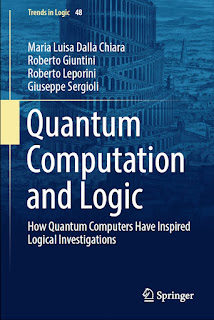QUANTUM PHYSICS

# Quantum Computation and Logic How Quantum Computers Have Inspired Logical Investigations PDF Free DownloadThis book provides a general survey of the main concepts, questions and results that have been developed in the recent interactions between quantum information, quantum computation and logic. Divided into 10 chapters, the books starts with an introduction of the main concepts of the quantum-theoretic formalism used in quantum information. It then gives a synthetic presentation of the main “mathematical characters” of the quantum computational game: qubits, quregisters, mixtures of quregisters, quantum logical gates. Next, the book investigates the puzzling entanglement-phenomena and logically analyses the Einstein–Podolsky–Rosen paradox and introduces the reader to quantum computational logics, and new forms of quantum logic.